Excel Tips & Tutorials:
Correlation and Bivariate Regression
 Suggest a Link Alphabetize Page Printer-Friendly List
Watch and Learn
Watch and Learn
Watch and Learn
Watch and Learn
 Building a Correlation Matrix with Data Analysis ToolPak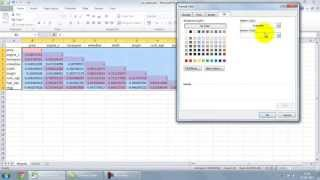Demo features car data (9:09)How to do Linear Regression with Data Analysis Toolpak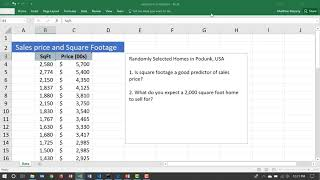Matt Macarthy demonstrates bivariate regression with home sale data(10:40).How To Make a Scatterplot with Slope, Y Intercept & R Value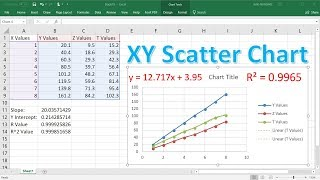Emphasizes Excel functions, another approach to regression analysis (13:23).Creating Correlation Table Using Data Analysis Tools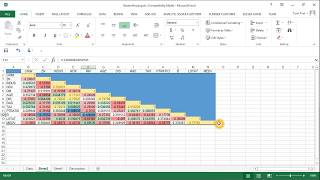Example based on Boston housing data, shows how to color-code results (9:39).Building a Correlation Matrix with Data Analysis ToolPakDemo features car data (9:09)How to do Linear Regression with Data Analysis ToolpakMatt Macarthy demonstrates bivariate regression with home sale data(10:40).How To Make a Scatterplot with Slope, Y Intercept & R ValueEmphasizes Excel functions, another approach to regression analysis (13:23).Building a Correlation Matrix with Data Analysis ToolPakDemo features car data (9:09)How to do Linear Regression with Data Analysis ToolpakMatt Macarthy demonstrates bivariate regression with home sale data(10:40).Building a Correlation Matrix with Data Analysis ToolPakDemo features car data (9:09)Regression Analysis Output Explained Page describes tables generated by Data Analysis Regression Tool in detail.Linear Regression Reference Real Statistics Using Excel shows how to conduct linear regression analysis, including advanced models.Regression Analysis Output Explained Page describes tables generated by Data Analysis Regression Tool in detail.Linear Regression Reference Real Statistics Using Excel shows how to conduct linear regression analysis, including advanced models.Regression Analysis Output Explained Page describes tables generated by Data Analysis Regression Tool in detail.Linear Regression Reference Real Statistics Using Excel shows how to conduct linear regression analysis, including advanced models.Regression Analysis Output Explained Page describes tables generated by Data Analysis Regression Tool in detail.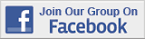Over 18,000 Members!